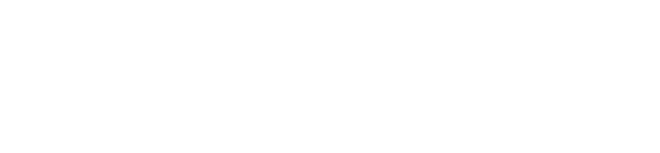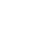# CLASSIFICATION of Inverters

Share

Home » CLASSIFICATION of Inverters

An inverter, is a power electronics device which used to convert fixed DC (Direct Current) into controlled AC (Alternating Current)”. Here, Controlled AC means we controlled two parameter of AC signal Frequency and Amplitude”.

## Classification Of Inverter

Inverter can be classified into many types based on their output, source, type of load etc.

(I) According to the Output Characteristic

1. Square Wave Inverter
2. Sine Wave Inverter
3. Modified Sine Wave Inverter

(II) According to the Source of Inverter

1. Current Source Inverter (CSI)
2. Voltage Source Inverter (VSI)

(III) According to the Type of Load

1. Single Phase Inverter
a) Half Bridge Inverter b) Full Bridge Inverter
1. Three Phase Inverter
a) 180-degree mode Inverter b) 120-degree mode Inverter

IV) According to different PWM Technique

1. Single Pulse Width Modulation (SPWM)
2. Multiple Pulse Width Modulation (MPWM)
3. Sinusoidal Pulse Width Modulation (SPWM)
4. Modified sinusoidal Pulse Width Modulation (MSPWM)

(V) According to the connection

1. Series Inverter
2. Parallel Inverter

One-by-one here i explain all the types of inverter –

(I) According to the Output Characteristic

There are three most common types of inverters used for powering AC loads include:

(1) pure sine wave inverter used for general applications (2) modified square wave inverter used for resistive, capacitive, and inductive loads and (3) square wave inverter used for some resistive loads.

ii) According to the Source of Inverter

According to the source there are two types of inverters

1. VSI (Voltage Source Inverter)– In voltage source inverter input voltage is kept constant. In this type of inverter DC voltage source having small or negligible impedance at the inverter input terminals. The waveform of the load current as well as its magnitude depends upon the nature of load impedance.

2.  CSI (Current Source Inverter) – In current source inverter input current is kept constant. CSI is fed with adjustable current source from a DC voltage source of high impedance. The magnitude of output voltage and its waveform depends upon the nature of the load impedance.

(III) According to the Type of Load

1. Single Phase Inverter– A single-phase inverter converts DC input into Single phase AC output. The output voltage/ output current of single-phase inverter has exactly one phase which has a nominal frequency of 50HZ or 60Hz a nominal voltage. Here Nominal voltage means voltage level at which electrical system operates. Single phase inverter is used for residential purpose (low loads).

There are two types of single-phase inverters –

a) full bridge inverter

b) half bridge inverter

• Three Phase Inverter- Three Phase inverter convert a DC voltage into a 3-ϕ AC supply. 3-ϕ inverters are most commonly used in industries than 1-ϕ inverters. Mostly, these types of inverters are used in high power applications and variable frequency drive applications like HVDC power transmission.

In a 3 phase, the power can be transmitted through the network with the help of three different currents all three are out of phase with each other, whereas in single-phase inverter, the power can transmit through a single phase.

There are two types of Three-phase inverters –

1. 180-degree conduction mode
2. 120-degree conduction mode

IV) According to different PWM Technique

Now a day mostly all inverters possess PWM technique. By using PWM technique we are capable of producing variable AC voltage.

PWM inverter has a wide range of applications. Practically these are used in power electronics circuits. The PWM inverter possess MOSFET’s in the switching stage of the output.

Types of PWM inverter-

1. Single Pulse Width Modulation (SPWM)
2. Multiple Pulse Width Modulation (MPWM)
3. Sinusoidal Pulse Width Modulation (SPWM)
4. Modified sinusoidal Pulse Width Modulation (MSPWM)

(V) According to the connection

According to the connection of component there are two types of inverters.

1. Series Inverter – In series inverter the commutating component L (inductor) and C (capacitor) connected in series with load RL (load resistance) that’s way it is called series inverter. Here L and C are called commutating component because they are responsible to turn off thyristors.

Series inverter is also known as a “self-commutated inverter” or “Load commutated inverter” because thyristors of this inverter circuit are commutated by itself from the load.

•  Parallel Inverter – In Parallel inverters the commutating component capacitor C comes in parallel with the load via the transformer that’s way it is called parallel inverter.  Parallel inverters are also known as center-tapped inverter because here we use a center-tapped transformer in between load and driving circuitry. Here the purpose of transformer is to change DC power into AC power of the required voltage.

What is inverter?

An inverter, is a power electronics device which used to convert fixed DC (Direct Current) into controlled AC (Alternating Current)”. Here, Controlled AC means we controlled two parameter of AC signal Frequency and Amplitude.

What are different types of inverters?

There are three most common types of inverters used for powering AC loads include:
(1) pure sine wave inverter used for general applications (2) modified square wave inverter used for resistive, capacitive, and inductive loads and (3) square wave inverter used for some resistive loads.

Which type of inverter is best for home?

Pure Sine Wave Inverter is the best for home.

What is KVA in inverter?

UPS systems are often “sized” by a kW (kilowatts) and/or a kVA (kilo-volt-amperes) rating. For example, a 1 kVA UPS means its circuitry can handle 1,000 volt-amperes. … For example, 1kVA UPS from N1C has the capacity to power 900 watts of connected equipment. This means the UPS has a “power factor” of 0.9.

Who Invent the Inverter?

Before inverter, DC (Direct Current) to AC (Alternating Current) conversion is done with the help of a motor-generator set and rotary converter. The term inverter was first introduced by David Prince titled “The Inverter” in 1925. Prince defined the inverter as the “Inverse of a Rectifier”.

Share

1.Biswajit Rajbanshi says:
2.SHASHICHAND PRASAD GUPTA says: